Question Pool Chemical reaction of organic compounds

# Chemical reaction of organic compounds - SAMAGRA Question Pool & Answers | Class 10 English Medium

Kerala Syllabus SAMAGRA SCERT SAMAGRA Question Pool for Class 10 English Medium Chemistry Chemical reaction of organic compoundsQn 1.

CH4 + Cl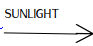A + HCl

a. What is the name of the compound A?

b. To which type does this reaction belong?

(Addition reaction, Substitution reaction, Combustion, Polymerisation‍)

a. CH3Cl

b. Substitution reaction

Qn 2.

Answer the question by analysing the equation given

1. CH4 + Cl→ A + HCl

2. CH4 + 2O2 → B + C+ heat

3. nCH2=CH2 → D

a) What are A, B, C and D

b)Name the product D formed during the third reaction

c)To which type of reaction does the first equation belong.?

a) A – CH3Cl

B – CO2

C - H2O

D –[CH2– CH2]n-

b. polythene

c. Substitution reaction

Qn 3.

Ethanol and Ester

A. CH3–COOH

B. CH3–CH2–OH

C. Petrol

Answer the questions related with the given compounds

a) Choose the substances which can be used to make an ester

b) Which of the above substances are used to make power alcohol?

a)CH3-COOH, CH3-CH2OH

b)CH3-CH2OH, Petrol

Qn 4.

Choose the suitable compounds from those given in brackets to make it undergo the following reaction

 CH4, C2H4, C8H18 , CH3Cl

1. Thermal cracking

2. Polymerisation

1. C8H18

2. C2 H4

Qn 5.

Why are hydrocarbons used as fuel ?

During the combustion of hydrocarbons large amount of heat energy is released /Burning of hydrocarbon is highly exothermic

Qn 6.

Uses of some organic compounds are given below.Choose the appropriate compound from the bracket according to each use.

(Teflon ‍, Ester‍, Ethanoic acid, ethanol‍, power alcohol)

1. Used as a solvent and in production of different organic compounds

2. Used in the production of artificial perfumes

3. Used as fuel in automobiles

4. Used in making  non-stick cooking vessels

1. ethanol‍,

2. Ester‍,

3. power alcohol

4. Teflon

Qn 7.

a) What is power alcohol?

b) What is its use ?

a)A mixture of absolute alcohol and petrol

b) It is used as fuel in automobiles

Qn 8.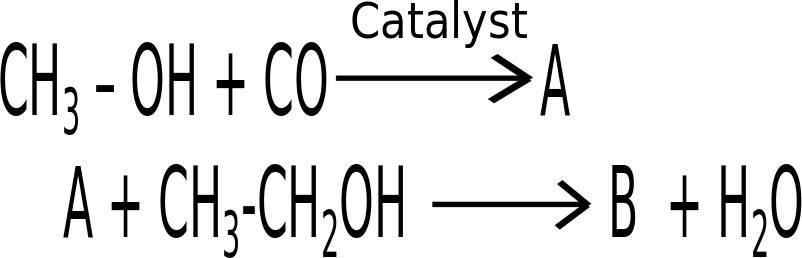1. Write the structures of A and B

2. Name the catagory of compounds to which B belongs?

1. A       CH3 COOH

B       CH3 COOCH2 - CH3

2. ester

Qn 9.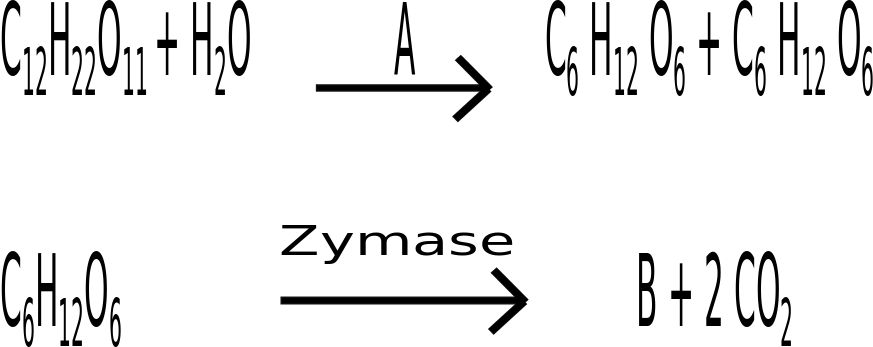1. what are A and B ?

2. How is rectified spirit produced from the mixture ?

1. A – Invertase . B – C2H5-OH

2. Fractional distillation

Qn 10.

Ethanol is produced by the fermentation of diluted molasses. What is meant by molasses?

Molasses is the concentrated solution of sugar left behind after separation of sugar crystals during the manufature of sugar

Qn 11.

C3H8 → CH4 + A

1. Give the molecular formula of A

2. To which catagory does the compound A belong?

(Alkane , Alkene ,Alkyne )

1. C2H4

2. Alkene

Qn 12.
1. What are the products formed by the complete burning of butane in air ?

2. Name this reaction.

1. CO2 , H2O (carbon dioxide, water)

2. Combustion.

Qn 13.

Which among the following can undergo addition reaction?

(C3H, C2H, CH, C4H10 )

C2 H4

Qn 14.

Match suitably

 Reactants Products Name of the reaction CH3–CH3 + Cl2 2CH3–CH3 + 7O2 CH2=CH2 + H2 CH3–CH2–CH3 CH2=CH2   + Cl2 CH3–CH3 4CO2 + 6H2O CH3–CH2–Cl + HCl Addition reaction Substitution reaction Thermal cracking Combustion

 Reactants Products Name of the reaction CH3–CH3 + Cl2 2CH3–CH3 + 7O2 CH2=CH2 + H2 CH3–CH2–CH3 CH3-CH2Cl + HCl 4CO2 + 6H2O CH3–CH3 CH2=CH2 + CH4 Substitution reaction Combustion Addition reaction Thermal cracking
Qn 15.

Give the products formed by the following reactions

1. CH2=CH2 + HCl → -------

2. CH≡CH + 2H→ ---------

a) CH3-CH2Cl

b) CH3-CH3

Qn 16.

An organic reaction is given

CH2=CH2 + A → CH3-CH2Cl

1. What is A?

2. What is the name for this type of reaction?

1. HCl

Qn 17.

An organic reaction is given.

A + Cl2 → CH3Cl + HCl

1. What is the compound A ?

2. By which name is this reaction known?

1. CH4

2. Substitution reaction

Qn 18.

An incomplete equation showing a polymerisation reaction is given

nCH2=CHCl → -----------

1. Draw the structrue of the product

2. Write any one use of the product

• PVC (structure).
• It is used for the production of pipes(or any other use)

Qn 19.

A polymerisation reaction is given

n CF2 = CF2 → -- [--CF2- CF2 --]-- n

a)Name the monomer .

b)What is the name of the polymer?

c)Give any one use of the polymer

a)Tetrafluoroethene

b)Teflon/ PTFE

c) It is used for coating on inner surface of non- stick cookware(Any other use)

Qn 20.

Examine the equation given

CH2=CH–CH3 + H2 → CH3–CH2–CH3

a) Choose the saturated hydrocarbon and unsaturated hydrocarbon in the above equation

b)By which name is this type of reactions are known?

a) Unsaturated hydrocarbon CH2=CH–CH3

Saturated hydrocarbon CH3–CH2–CH3

Qn 21.

See the equation given

CH3–COOH + CH3–OH → A + H2O

a) Write the formula of A and complete the equation.

b)To which catagory of compounds does A belong ?

a) CH3-COO-CH3

b) Esters

Qn 22.

a)How is ethanol converted to denatured spirit ?

b) Why is it necessary? Explain

a) By adding poisonous substance like methanol

b) ‍For preventing the use of industrial alcohol as beverage .

Qn 23.

Name the polymer formed by the combination of vinylchloride molecules

Poly vinylchloride/PvC

Qn 24.

Structural formula of some compounds are given

1. CH3–CH2–COO–CH3

2. CH3–CH2–COOH

3. CH3–CH2–CO-CH3

4. CH3–OH

a)Which  of the above  compunds represent an ester ?

b)Name the compounds needed to prepare this ester

c)Write the equation of the above reaction.

d)Write one use of ester.

a)CH3–CH2–COO–CH3

b)Methanol and ‍Propanoic acid or (CH3–OH, CH3–CH2–COOH )

c)CH3–CH2–COOH + CH3–OH → CH3-CH2-COO-CH+ H2O

d) To make sweet smell of flowers and fruits

Qn 25.

Some equations are given

CH2 = CH+ A → CH3 – CH3

CH3 – CH+ Cl2 → B + HCl

1. Fiind out A and B

2. Write the name of the second reaction

1. A   -    H2                               B -  CH3–CH2-Cl

2. Substitution reaction

Qn 26.

PVC is a polymer used for the preparation of pipes. What is the name of its monomer?

Vinyl chloride / CH= CHCl

Qn 27.

Choose the two products formed by the thermal cracking of CH3–CH2–CHfrom the box given below and write them.

 CH4                           CH3–CH2–CH=CH2 CH2=CH2                                      CH3–CH2-CH3

CH,CH2=CH2

Qn 28.

Choose Grape spirit and Wood spirit from the following

(CH3-CH2-OH, CH3-COOH, CH3-OH, )

Grape spirit - CH3-CH2-OH

Wood spirit - CH3-OH

Qn 29.

Formula of a compound is CH3–OH

1. Write the IUPAC name of the compound? 1

2. Write two uses of this compound

1. Methanol

2. Manufacture of many compounds such as formalin,antifreeze,denaturant e.t.c (Any two)

Qn 30.
1. Complete the following equation
nCH2=CHCl → ------

2. Write the name of the reaction

a)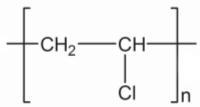b) Polymerisation

Qn 31.

Some compounds are given

A. CH= CH – CH2 – CH3

B. CH3 – CH2 – CH3

C. CH3 – CH = CH2

D. CH4

E. CH3 – CH2 – CH2 – CH3

1. Which of these compounds can form polymer?

2. What are the products obtained by thermal cracking of B?

1. CH2=CH–CH2-CH3, CH3-CH=CH2

2. CH2=CH2, CH4

Qn 32.

a) How is ethanoic acid prepared industrially?

b)Write any two uses of ethanoic acid.

a) Ethanoic acid can be manufactured by reacting methanol with carbon monoxide in the presence of catalyst

CH3-OH + CO → CH-COOH

b) In rayon,rubber and silk industries,for manufacture of vinegar.

Qn 33.

Ethanol is prepared from sugar solution by adding yeast.

a) What is the name of the process by which ethanol is produced ?

b) Which enzymes present in yeast  are used in this process?

1. Fermentation

2. Invertase, Zymase

Qn 34.

Explain the following

1. Wash

2. Rectified spirit

3. Absolute alcohol

a) 8-10% ethanol is known as wash

b)Wash is subjected to fractional distillation to get 95.6% ethanol , known as

rectified spirit

c) Ethanol of purity above 99% is known as absolute alcohol

Qn 35.

Write any two uses of ethanol

1. As preservative

2.   As  fuel (any two uses)

Qn 36.

Match the following

 A B CH2 = CH2 + H2 → CH3 – CH3 CH3 – CH2 – CH3 → CH2 = CH2 + CH4 CH4 + Cl2 → CH3Cl + HCl n CH2 = CH2 → [CH2 – CH2]n Polymerisation Substitution Additon reaction Thermal cracking

2. Thermal cracking

3. Substitution

4. Polymerisation

Qn 37.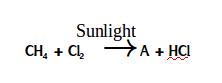a. What is the name of the compound A?

b. To which type does this reaction belong?

(Addition reaction, Substitution reaction, Combustion, Polymerisation‍) (1)

a. CH3Cl

b. Substitution reaction

Qn 38.

CH-CH = CH2 + Cl2 → A

a which is the reactant hydrocarbon?

b. Write the name or structure of the product A

c. Write the name of the reaction

a. CH3 – CH = CH/ Propene

b. CH– CHCl– CH2Cl / 1,2-dichloro propane.

Qn 39.

A  CH ≡ CH + H →X

B   X + H2 →Y

C   Y + Cl2 →Z + HCl

a. Write the molecular formula of the compounds X, Y and Z

b. By which name the reaction B is known?

a) X. CH2 = CH2

Y. CH3 – CH3

Z. CH3 – CH2Cl

Qn 40.

Different types of reactions are given in brackets‍

( Addition reaction, Polymerisation, ‍ Substitution, Combustion )

a. CH+ 202  →CO+ 2 H2O

b. CH– CH+ Cl2 →CH– CH2 – Cl + HCl

c. n CH2 = CH →- -[- CH2-CH2--]-n

d. CH2 = CH+ H2 →CH3 – CH3

Find the type of reaction to which each of the above ones belong and write them.

a. Combustion

b. Substitution

c. Polymerisation

Qn 41.

CH2 = CH2 + HCl →X

a. Write the chemical formula of the product X formed during the reaction

b. Write the IUPAC name of the product

a.CH3-CH2Cl

b. Chloroethane

Qn 42.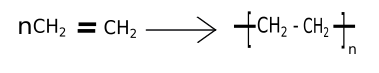a. Which is the monomer of this reaction?

b. Write the name of the polymer.

c. Which type of chemical reaction is this?